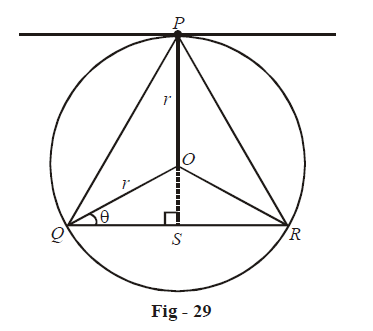# Examples on Maxima and Minima Set 5

Go back to  'Applications of Derivatives'

Example – 29

A point P is given on the circumference of a circle of radius r. The chord QR is parallel to the tangent line at P. Find the maximum area of $$\Delta PQR$$.

Solution: Observe that since QR is parallel to the tangent at P, the triangle PQR must be isosceles. This will become more clear upon carefully observing the following figure:We can assume either $$\angle OQS$$ or the length OS as the variable on which the area A will be dependent. We let the variable be$$\angle OQS = \theta$$.

\begin{align} &\Rightarrow \qquad OS = r\sin \theta \\\\&\Rightarrow \qquad PS = r + r\sin \theta \\ \text{and}\;QR &= 2r cos \theta\\\\&\Rightarrow \qquad A = \frac{1}{2} \times QR \times PS\\\\ & \qquad \qquad= {r^2}\cos \theta \left( {1 + \sin \theta } \right)\end{align}

\begin{align}\text{For maximum area,} \qquad \frac{{dA}}{{d\theta }} &= 0\;\text{and}\;\frac{{{d^2}A}}{{d{\theta ^2}}} < 0\\\\ \frac{{dA}}{{d\theta }} &= {r^2}\left\{ { - \sin \theta \left( {1 + \sin \theta } \right) + \cos \theta .\cos \theta } \right\}\\\\ &= {r^2}\left\{ {{{\cos }^2}\theta - {{\sin }^2}\theta - \sin \theta } \right\}\\\\ &= {r^2}\left\{ {1 - \sin \theta - 2{{\sin }^2}\theta } \right\}\\\\&= {r^2}\left( {1 + \sin \theta } \right)\left( {1 - 2\sin \theta } \right)\end{align}

\begin{align}&\text{This is 0 when} \qquad \qquad \sin \theta = \frac{1}{2} \Rightarrow \,\,\,\theta = \frac{\pi }{6}\\\\&\quad\qquad\qquad\qquad\qquad\text{or sin} \theta = \,–1 \qquad \text{(not a possible case)}\\&\text{Verify that}\; \frac{{{d^2}A}}{{d{\theta ^2}}} < 0 \;\; \text{for}\;\; \theta = \frac{\pi }{6}\\\\&\text{Therefore, area is maximum for}\;\theta = \frac{\pi }{6}.\\&\qquad \qquad \qquad {\left. A_\max= {r^2}\cos \theta \left( {1 + \sin \theta } \right) \right|_{\theta = \pi /6}}\\\\ &\qquad \qquad \qquad \qquad \;= \frac{{3\sqrt 3 \,{r^2}}}{4}\end{align}

Example - 30

Find the point on  the curve $$4{x^2} + {a^2}{y^2} = {\rm{ }}4{a^2},{\rm{ }}4{\rm{ }} < {a^2} < {\rm{ }}8$$, that is farthest from the point $$\left( {0,{\rm{ }}-2} \right).$$

Solution: Upon rearrangement, the equation of the curve gives \begin{align}\frac{{{x^2}}}{{{a^2}}} + \frac{{{y^2}}}{4} = 1\end{align}  which is the equation of an ellipse. A general variable point P on this ellipse can be taken as (a cos$$\theta$$, 2 sin$$\theta$$).

Let r represent the distance of P from (0, –2).

\begin{align}{r^2} &= {{\rm{ }}{{\left( {acos\theta {\rm{ }}-0} \right)}^2} + {\rm{ }}{{\left( {2{\rm{ }}sin\theta + {\rm{ }}2} \right)}^2}}\\\\&= {{a^2}co{s^2}\theta {\rm{ }} + {\rm{ }}4{\rm{ }}{{\left( {1{\rm{ }} + {\rm{ }}sin{\rm{ }}\theta } \right)}^2}} \end{align}

For $${r^2}$$ to be maximum, \begin{align}\frac{{d\left( {{r^2}} \right)}}{{d\theta }} = 0\end{align}  and \begin{align}\frac{{{d^2}\left( {{r^2}} \right)}}{{d{\theta ^2}}} < 0\end{align}

$\frac{{d\left( {{r^2}} \right)}}{{d\theta }} = - 2{a^2}\sin \theta \cos \theta + 8\left( {1 + \sin \theta } \right)\cos \theta$

$= \cos \theta \left\{ {\left( {8 - 2{a^2}} \right)\sin \theta + 8} \right\}$

This is 0 when:

\begin{align}&\text{cos}\,\theta = 0 \qquad \Rightarrow \qquad \theta = \frac{\pi }{2}\\&\qquad \qquad \qquad\text{or}\\&(8{\rm{ }}-{\rm{ }}2{a^2}){\rm{ }}\,\text{sin}\;\theta = {\rm{ }}-{\rm{ }}8 \Rightarrow \,\,\sin \theta = \frac{4}{{{a^2} - 4}}\end{align}

But \begin{align}\frac{4}{{{a^2} - 4}} > 1\end{align}  (verify) and sin$$\theta$$  cannot be greater than 1. Hence, this case does not given any valid value of  $$\theta$$.

$\Rightarrow \,\theta = \frac{\pi }{2}\\\text{Verify that}\; {\left. {\frac{{{d^2}\left( {{r^2}} \right)}}{{d{\theta ^2}}}} \right|_{\theta = \pi /2}} < 0\\ \Rightarrow \theta = \frac{\pi }{2} \text{ is a local maximum for } r^2$

The required point is \begin{align}{\left. {\left( {a\cos \theta ,2\sin \theta } \right)} \right|_{\theta = \pi /2}}\end{align} or $$\left( {0,{\rm{ }}2} \right).$$

TRY YOURSELF - IV

Q. 1     Find the minimum distance of a point on the curve \begin{align}\frac{{{a^2}}}{{{x^2}}} + \frac{{{b^2}}}{{{y^2}}} = 1\end{align} from the origin.

Q. 2     For a given curved surface area of a right circular cone, find the semi-vertical angle for which the volume of the cone is maximum.

Q. 3     Find the local maxima/minima values for the following funtions:

(a) \begin{align}\frac{{40}}{{3{x^4} + 8{x^3} - 18{x^2} + 60}}\end{align}

(b) \begin{align}\frac{x}{{1 + x\tan x}}\end{align}

(c) \begin{align}2x - {\tan ^{ - 1}}x - \log \left( {x + \sqrt {1 + {x^2}} } \right)\end{align}

(d) \begin{align}x{\left( {\log x} \right)^2}\end{align}

(e) \begin{align}{x^2}\log x\;{\rm{on}}\;[1,e]\end{align}

(h) \begin{align}\sqrt {\left( {1 - {x^2}} \right)\left( {2{x^2} + 1} \right)} \;{\rm{on}}\;\left[ { - 1,\;1} \right]\end{align}

Q. 4     A point P is given on the circumference of a circle of radius r. The chord QR is parallel to the tangent at P. Find the maximum area of $$\Delta PQR$$

Q. 5     A chord of length 2l divides a circular area of radius r into two segments. Find the sides of the rectangle with the largest area that can be inscribed in the smaller segment.